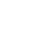# 风道设计计算的方法与步骤(带例题）

1．假定流速法

2．压损平均法

3．静压复得法

1．确定空调系统风道形式，合理布置风道，并绘制风道系统轴测图，作为水力计算草图。

2．在计算草图上进行管段编号，并标注管段的长度和风量。

3．选定系统最不利环路，一般指最远或局部阻力最多的环路。

4．选择合理的空气流速。5．根据给定风量和选定流速，逐段计算管道断面尺寸，然后根据选定了的风管断面尺寸和风量，计算出风道内实际流速。

6．计算风管的沿程阻力

7．计算各管段局部阻力

8．计算系统的总阻力，△P=∑（△pyl +△Pj）。

9．检查并联管路的阻力平衡情况。

10．根据系统的总风量、总阻力选择风机。、

\某公共建筑直流式空调系统，如图所示。风道全部用镀锌钢板制作，表面粗糙度K=0.15mm。已知消声器阻力为50Pa，空调箱阻力为290 Pa，试确定该系统的风道断面尺寸及所需风机压头。[解

1．绘制系统轴测图，并對各管段进行编号，标注管段长度和风量。

2．选定最不利环路，逐段计算沿程压力损失和局部压力损失。本系统选定管段1—2—3—4—5—6为最不利环路。

3．列出管道水力计算表8-4，并将各管段流量和长度按编号顺序填入计算表中。

4．分段进行管道水力计算,并将结果均列入计算表8-4中。

F’=1500/(3600×4)=0.104m2

υ=1500/（3600×0.102）=4.08m/s根据流速4.08m/s，查附录13，得到单位长度摩擦阻力△py=0.7Pa/m,则管段1—2的沿程阻力：

△Py=△py×l=0.7×9=6.3Pa

υ=1500/（3600×0.6×0.6）=1.16m/s根据面风速1.16m/s和开孔率0.3，查附录14序号35，得孔板局部阻力系数ζ=13，故孔板的局部阻力

△pj1=13×(1.2×1.162)/2=10.5Pa渐扩管：渐扩管的扩张角α=22.5°，查附录14序号4，得ζ=0.6，渐扩管的局部阻力

△pj2=0.9×(1.2×4.082)/2=5.99Pa多叶调节阀：根据三叶片及全开度，查附录14序号34，得ζ=0.25，多叶调节阀的局部阻力

△pj3=0.25×(1.2×4.082)/2=2.5Pa弯头：根据α=90°，R/b=1.0，查附录14序号9，得ζ=0.23，弯头的局部阻力

△pj4=0.23×(1.2×4.082)/2=2.3Pa渐缩管：渐缩管的扩张角α=30°<45°,查附录14序号7，得ζ=0.1，渐缩管的局部阻力

△pj5=0.1×(1.2×4.082)/2=1Pa直三通管：根据直三通管的支管断面与干管断面之比为0.64，支管风量与总风量之比为0.5，查附录14序号19，得ζ=0.1，则直三通管的局部阻力

△Pj6=0.1×(1.2×5.22)/2=1.6Pa （取三通入口处流速）

=10.5+5.99+2.5+2.3+1+1.6

=23.89Pa该管段总阻力

△P1-2=△Py+△Pj=6.3+23.89=30.19Pa

△Py=△py×l=0.8×5=4.0Pa

△Pj =0.28×(1.2×6.252)/2= 6.6Pa. （取总流流速）

△Py=△py×l=0.96×9=8.64Pa局部压力损失计算：该管段存在局部阻力的部件有消声器、弯头、风量调节阀、软接头以及渐扩管。

△pj1= 50.0Pa

△pj2=0.2×(1.2×6.252)/2=4.7Pa

△pj3=0.25×(1.2×6.252)/2=5.9Pa软接头：因管径不变且很短，局部阻力忽略不计。

υ=4500/（3600×0.315×0.36）=11m/s

△Pj=△pj1+△pj2+△pj3+△pj4

=50.0+4.7+5.9+10.9

=71.5Pa

△P3-4=△Py+△Pj=8.64+71.5=80.14Pa管段4—5：

△P4-5=△Pj=290Pa

△Py=△py×l=0.96×6=5.76Pa

△pj1=0.64×(1.2×6.252)/2=15.1Pa弯头(两个)：根据α=90°，R/b=1.0，a/b=0.8,查附录14序号10，得ζ=0.20，弯头的局部阻力

△pj2=0.2×(1.2×6.252)/2=4.7Pa

2△pj2=4.7×2=9.4 Pa

υ=6.25m/s 渐缩管的局部阻力

△pj3=0.1×(1.2×6.252)/2=2.36Pa

0.63×0.5×0.8=0.252m2

△p4=0.9×(1.2×52)/2=13.5Pa

=15.1+9.4+2.36+13.5

=40.36Pa

△P5-6=△Py+△Pj=5.76+40.36=46.12Pa5．检查并联管路的阻力平衡

△P8-2=△Py+△Pj=1.4+25.8=27.2Pa检查并联管路的阻力平衡：

（△P1-2-△P8-2）/△P1-2=（30.19-27.2）/30.19=9.9%<15%

（△P1-2-3-△P7-3）/△P1-2-3=（40.79-38）/40.79=6.8%<15%

=30.19+10.6+80.14+290+46.12

=457.05 Pa△P=△pyl（1+k）+∑△ps (Pa)

l—最不利环路总长度，即到最远送风口的送风管总长度加上到最远回风口的回风管总长度，m。

k—局部压力损失与沿程压力损失之比值：

∑△ps—考虑到空气通过过滤器、喷水室（或表冷器）、加热器等空调装置的压力损失之和。• 收藏0

• 打赏0

• 2019年09月27日 09:07:52 2楼

感谢分享

回复 举报 点赞
• 2019年09月27日 10:44:04 4楼

楼主辛苦。谢谢分享！

回复 举报 点赞
• 2019年09月28日 11:05:18 6楼

谢谢分享

回复 举报 点赞
• 2019年09月29日 08:23:35 7楼

楼主辛苦。谢谢分享！

回复 举报 点赞
• 2019年09月29日 08:51:38 8楼

感谢分享，谢谢楼主

回复 举报 点赞
• 2019年09月29日 09:16:04 9楼

楼主辛苦。谢谢分享!!!

回复 举报 点赞
• 2019年11月21日 16:57:12 11楼

谢谢楼主的资料

回复 举报 点赞##### TA的关注